# ISEE Middle Level Quantitative : How to add variables

## Example Questions

2 Next →

### Example Question #11 : Algebraic Concepts

Define an operation on the real numbers as follows:

For all real values ofand,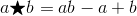is a positive number. Which is the greater quantity?

(a)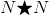(b)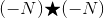(b) is the greater quantity

(a) and (b) are equal

(a) is the greater quantity

It is impossible to determine which is greater from the information given

(a) and (b) are equal

Explanation:so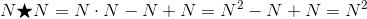and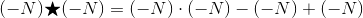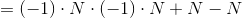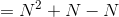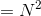The two are equal regardless of the value of.

### Example Question #12 : Algebraic Conceptsandare both negative numbers. Which is the greater quantity?

(a)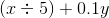(b)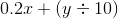(b) is the greater quantity

(a) and (b) are equal

(a) is the greater quantity

It is impossible to determine which is greater from the information given

(a) and (b) are equal

Explanation:

The two quantities are equal regardless of the values ofand. To see this, we note that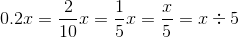and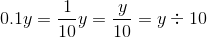Therefore, by the addition property of equality,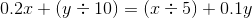### Example Question #13 : Algebraic Concepts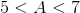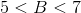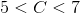Which is the greater quantity?

(a)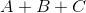(b) 18

(a) is the greater quantity

(a) and (b) are equal

It is impossible to determine which is greater from the information given

(b) is the greater quantity

It is impossible to determine which is greater from the information given

Explanation:

The information is insufficient, as we see by exploring two cases:

Case 1: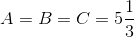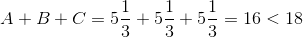Case 2: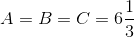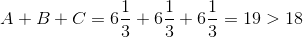Remember, the three variables need not stand for whole numbers.

2 Next →